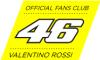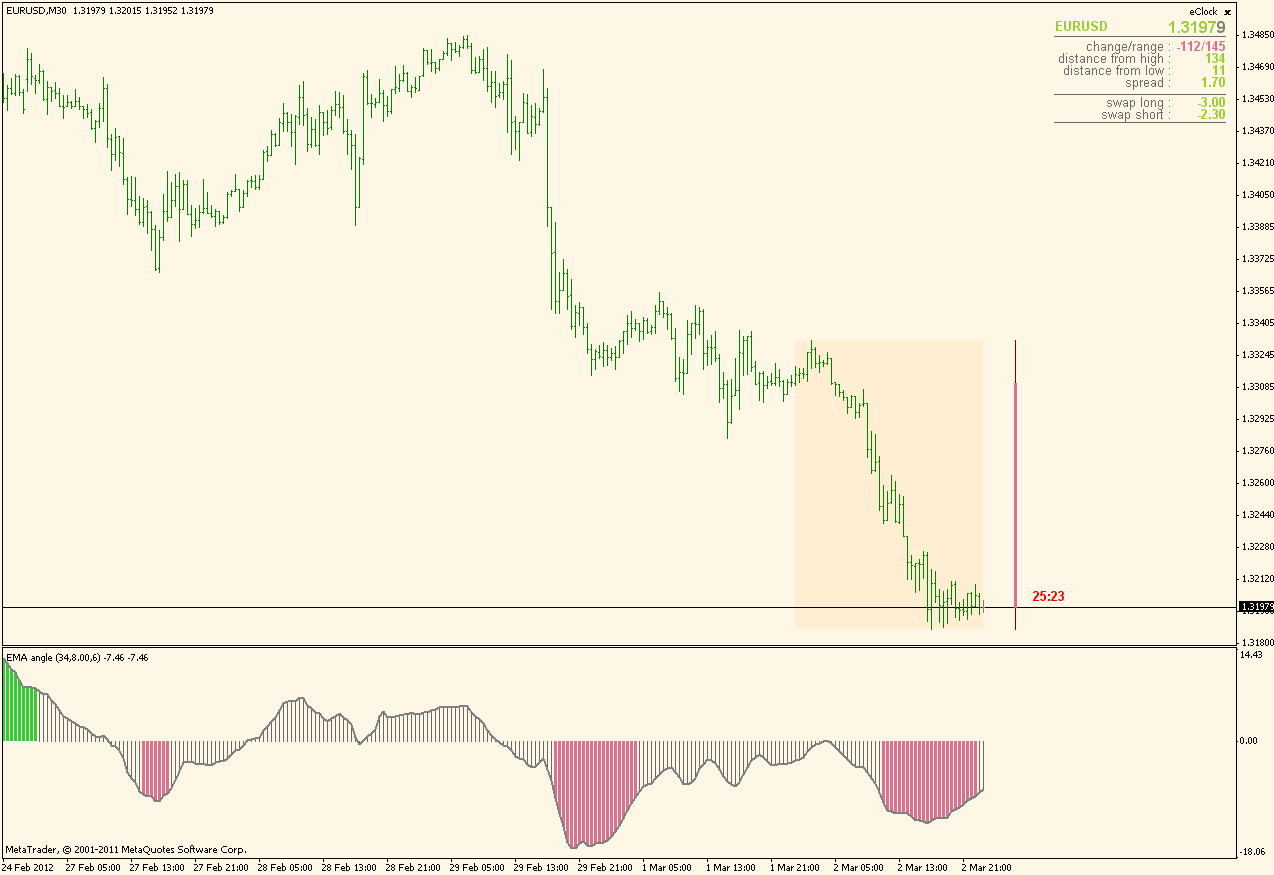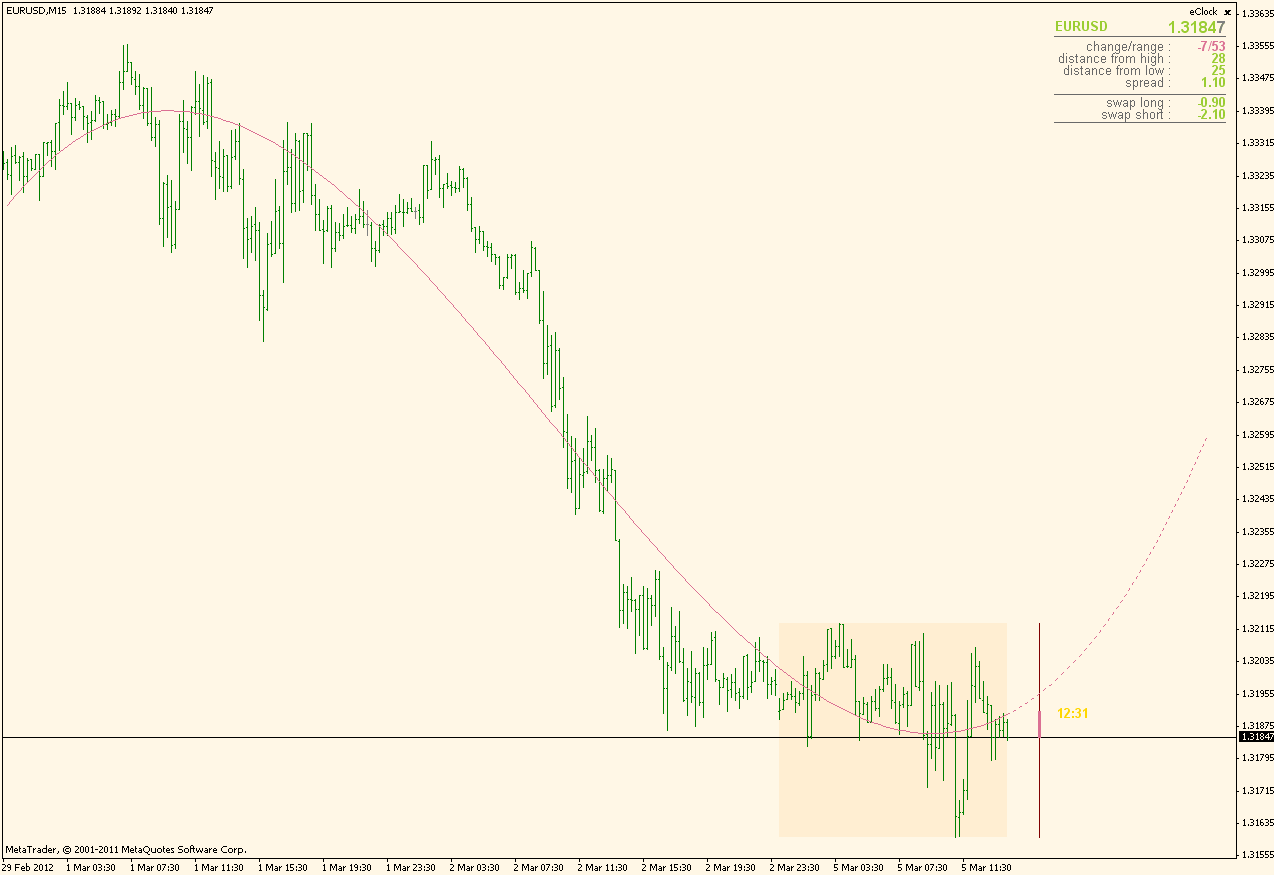# Requests & Ideas - page 115158

Hi Simba

thaks a lot for your precious input; I didn't apply your method yet because I was still testing the first idea that I had to weight the time frames as follow:

if(Period() == PERIOD_M1){

TST = (TS0 + TS1*1/5 + TS2*1/15 + TS3*1/30 + TS4*1/60 + TS5*1/240 + TS6*1/1440 + TS7*1/10080);

coeff = ( 6 + 6*1/5 + 6*1/15 + 6*1/30 + 6*1/60 + 6*1/240 + 6*1/1440 + 6*1/10080);}

if(Period() == PERIOD_M5){

TST = (TS0*1/5 + TS1 + TS2*1/3 + TS3*1/6 + TS4*1/12 + TS5*1/48 + TS6*1/288 + TS7*1/2016);

coeff = ( 6*1/5 + 6 + 6*1/3 + 6*1/6 + 6*1/12 + 6*1/48 + 6*1/288 + 6*1/2016);}

if(Period() == PERIOD_M15){

TST = (TS0*1/15 + TS1*1/3 + TS2 + TS3*1/2 + TS4*1/4 + TS5*1/16 + TS6*1/64 + TS7*1/672);

coeff = ( 6*1/15 + 6*1/3 + 6 + 6*1/2 + 6*1/4 + 6*1/16 + 6*1/64 + 6*1/672);}

if(Period() == PERIOD_M30){

TST = (TS0*1/30 + TS1*1/6 + TS2*1/2 + TS3 + TS4*1/2 + TS5*1/8 + TS6*1/32 + TS7*1/336);

coeff = ( 6*1/30 + 6*1/6 + 6*1/2 + 6 + 6*1/2 + 6*1/8 + 6*1/32 + 6*1/336);}

if(Period() == PERIOD_H1){

TST = (TS0*1/60 + TS1*1/12 + TS2*1/4 + TS3*1/2 + TS4 + TS5*1/4 + TS6*1/24 + TS7*1/168);

coeff = ( 6*1/60 + 6*1/12 + 6*1/4 + 6*1/2 + 6 + 6*1/4 + 6*1/24 + 6*1/168);}

if(Period() == PERIOD_H4){

TST = (TS0*1/240 + TS1*1/48 + TS2*1/16 + TS3*1/8 + TS4*1/4 + TS5 + TS6*1/6 + TS7*1/42);

coeff = ( 6*1/240 + 6*1/48 + 6*1/16 + 6*1/8 + 6*1/4 + 6 + 6*1/6 + 6*1/42);}

if(Period() == PERIOD_D1){

TST = (TS0*1/1440 + TS1*1/288 + TS2*1/96 + TS3*1/48 + TS4*1/24 + TS5*1/6 + TS6 + TS7*1/7);

coeff = ( 6*1/1440 + 6*1/288 + 6*1/96 + 6*1/48 + 6*1/24 + 6*1/6 + 6 + 6*1/7);}

if(Period() == PERIOD_W1){

TST = (TS0*1/10080 + TS1*1/2016 + TS2*1/672 + TS3*1/336 + TS4*1/168 + TS5*1/42 + TS6*1/7 + TS7);

coeff = ( 6*1/10080 + 6*1/2016 + 6*1/672 + 6*1/336 + 6*1/168 + 6*1/42 + 6*1/7 + 6);}

percent = MathRound(TST / coeff * 100);

where "TST" is the total trend score and the "coeff" is the maximum value that the TST can reach, needed to determinate the percentage; my "n" is +6/-6. Basically I'm assuming 100% the trend score of the active time frame and assign the value for the others multiplied by 1/bars.

Honestly I don't know if this approach is baked by a "mathematical mean", but seems to work not really bad; visually I can see that the indicator is quite responsive in the lowest time frames and smooth in the highest.

During the weekend I'll try to apply your concept with the 2 options you proposed and I'll let you know how it works.

Best Regards

brax

SIMBA:
Brax,

Not that I know.

Please notice that while the weight is biased,as it should, towards the higher tfs,the concept may allow enough responsiveness provided by the lower tfs.

.....

....

...

S158

Moving Averages 3.0 aka NMA...

after reading the last IFTA journal (thanks for the link!), the article about this new type of average stimulate my curiosity; without rush could you please have a look at that and create the indicator? Also seem interesting the applications based on this new type of averages (the digitized Aroon oscillator and the Stochastic-RSI applied to a NWMA).

Thank a zillion for your help

Have a nice weekend!

brax

Files:
ma3.0.pdf 1343 kb49

Can any please refer to to an indicator that uses "angle", or pass onto me a routine for "angle" of an indicator.

I would really appreciate it.

Regards

gorick162715

...

gorick

There can not be an angle calculation on a time series charts. The problem is that you have one measurement unit on the x axis (time) and a completely different measurement unit on the y axis (value). Hence there can not be an angle of something on a time series chart. It can only be something called "slope over time" or there can be an approximation of some kind. The approximation I use is converting time into ATR (meaning that horizontally one bar is "worth" an average true range points - that way if the "vertical" change on that bar is that equal number of points the angle is 45 degrees, and so on, but in that case those are comparable values and they even give some reasonable (logical) results)

Attaching an indicator that uses that logic (it was attached in the "Elite indicators" thread too) so you can check it. Also, using atr to convert time to units similar to chart values is limiting its usage (it can be used on moving averages and similar ones, but can not be used on oscillators like stochastic or rsi for example). Here is how it looks when converted to atr and then an angle is calculated (there will be no ilogical results like angles over 90 for example)regards

gorick:
Can any please refer to to an indicator that uses "angle", or pass onto me a routine for "angle" of an indicator.

I would really appreciate it.

Regards

gorick
Files:49

gorick

There can not be an angle calculation on a time series charts. The problem is that you have one measurement unit on the x axis (time) and a completely different measurement unit on the y axis (value). Hence there can not be an angle of something on a time series chart. It can only be something called "slope over time" or there can be an approximation of some kind. The approximation I use is converting time into ATR (meaning that horizontally one bar is "worth" an average true range points - that way if the "vertical" change on that bar is that equal number of points the angle is 45 degrees, and so on, but in that case those are comparable values and they even give some reasonable (logical) results)

Attaching an indicator that uses that logic (it was attached in the "Elite indicators" thread too) so you can check it. Also, using atr to convert time to units similar to chart values is limiting its usage (it can be used on moving averages and similar ones, but can not be used on oscillators like stochastic or rsi for example). Here is how it looks when converted to atr and then an angle is calculated (there will be no ilogical results like angles over 90 for example)regards

Thank you for your input, you are right in your observations and explanations. I did mean something tha gives what you have provided thank you.

I also will ask if there is something available that can replace multiple lines with a single line approximation? A line of best fit, or a regression? I am asking this as it goes back to my question of looking for a sideways market.

Regards

gorick49

gorick

There can not be an angle calculation on a time series charts. The problem is that you have one measurement unit on the x axis (time) and a completely different measurement unit on the y axis (value). Hence there can not be an angle of something on a time series chart. It can only be something called "slope over time" or there can be an approximation of some kind. The approximation I use is converting time into ATR (meaning that horizontally one bar is "worth" an average true range points - that way if the "vertical" change on that bar is that equal number of points the angle is 45 degrees, and so on, but in that case those are comparable values and they even give some reasonable (logical) results)

Attaching an indicator that uses that logic (it was attached in the "Elite indicators" thread too) so you can check it. Also, using atr to convert time to units similar to chart values is limiting its usage (it can be used on moving averages and similar ones, but can not be used on oscillators like stochastic or rsi for example). Here is how it looks when converted to atr and then an angle is calculated (there will be no ilogical results like angles over 90 for example)regards

Would it be possible to have a version of the "angle of average" applied to the each of "jurik simple 1" and also OMA?

gorick9493

gorick:

Would it be possible to have a version of the "angle of average" applied to the each of "jurik simple 1" and also OMA?

gorick

Hi Gorick,

This the Angles of jurik and Oma averages,on the jurik, for jurik filter simple just leave smooth double = false and on the Oma the angle levels need to be adjusted lower.49

mrtools:
Hi Gorick, This the Angles of jurik and Oma averages,on the jurik, for jurik filter simple just leave smooth double = false and on the Oma the angle levels need to be adjusted lower.

Thank you Mr Tools.162715

...

Of regression : did you try "Regression channel" (from this post : https://www.mql5.com/en/forum/general or from this one : https://www.mql5.com/en/forum/general ) and set the deviations to 0. That way you are can get a single line display (that way it might be what you are looking for - it looks like the example bellow)gorick:

Thank you for your input, you are right in your observations and explanations. I did mean something tha gives what you have provided thank you.

I also will ask if there is something available that can replace multiple lines with a single line approximation? A line of best fit, or a regression? I am asking this as it goes back to my question of looking for a sideways market.

Regards

gorick
Files:51